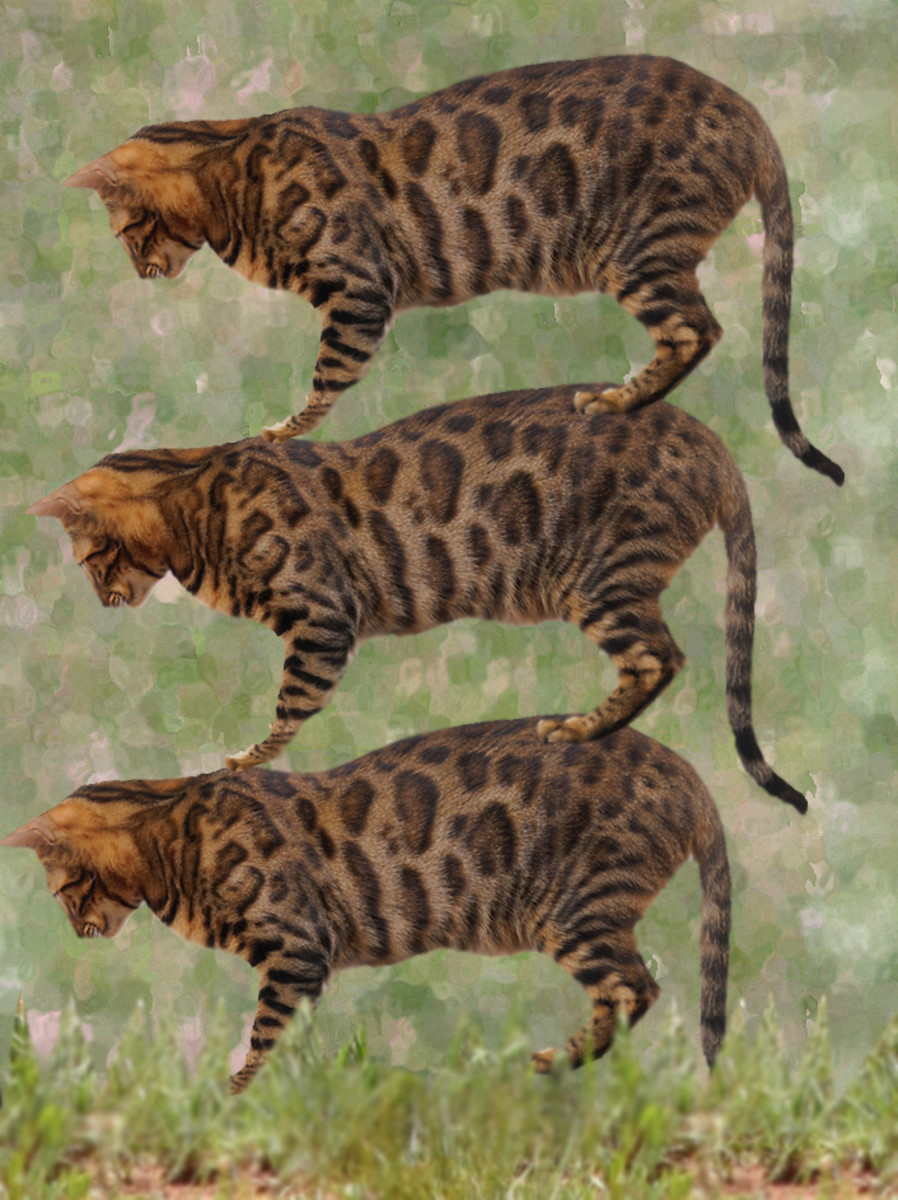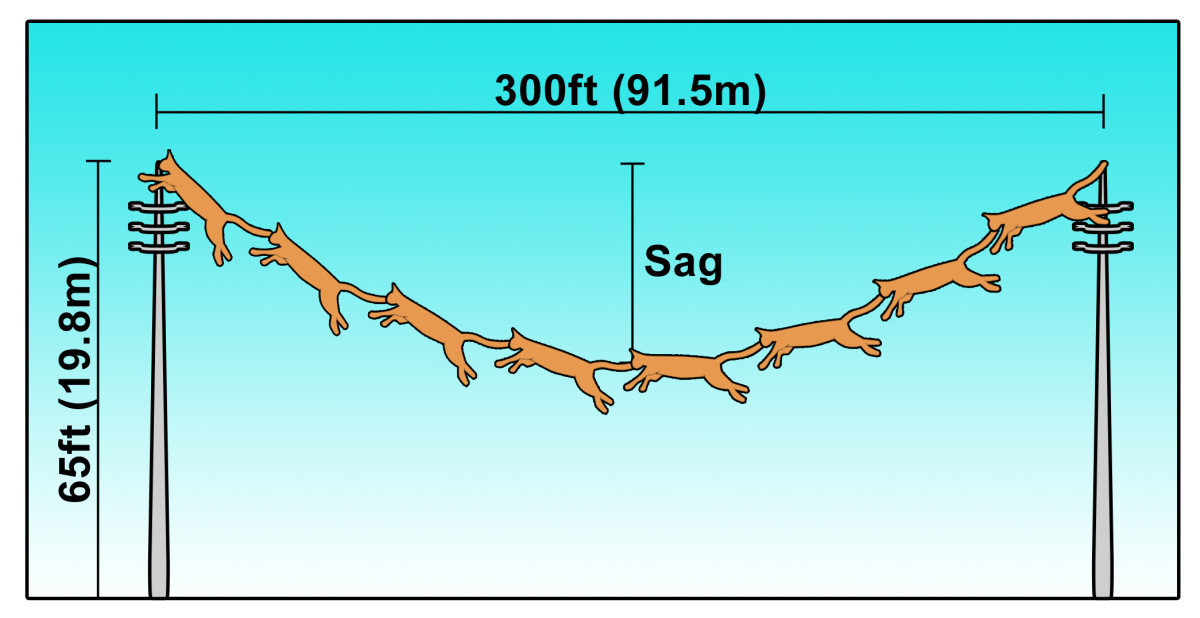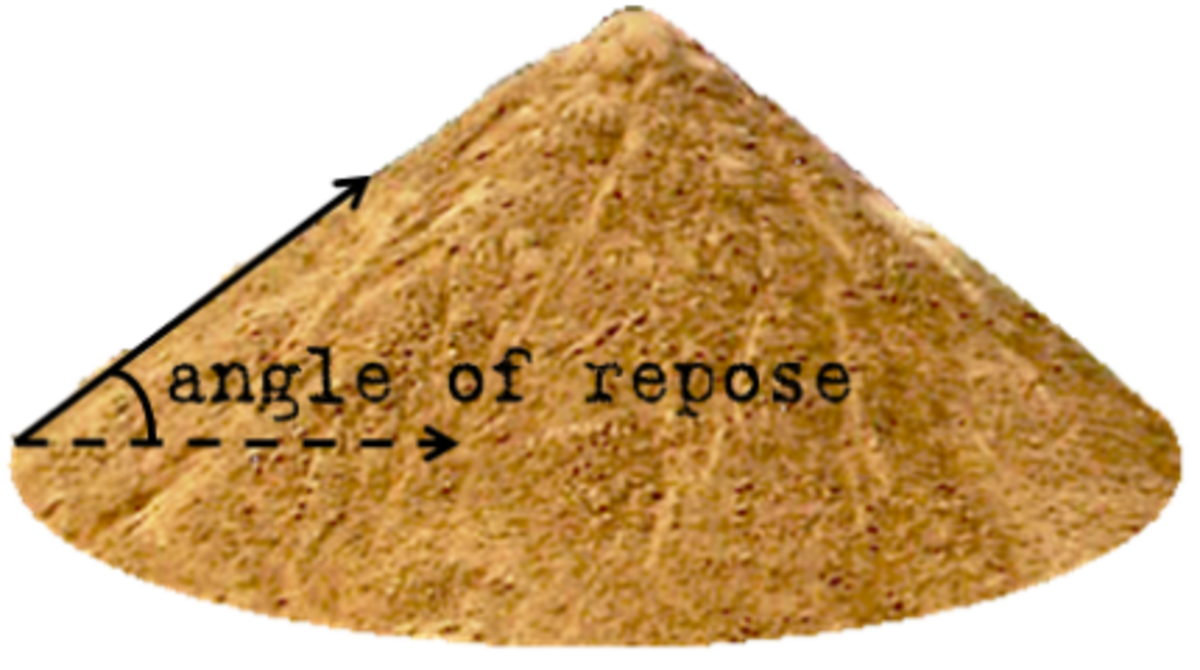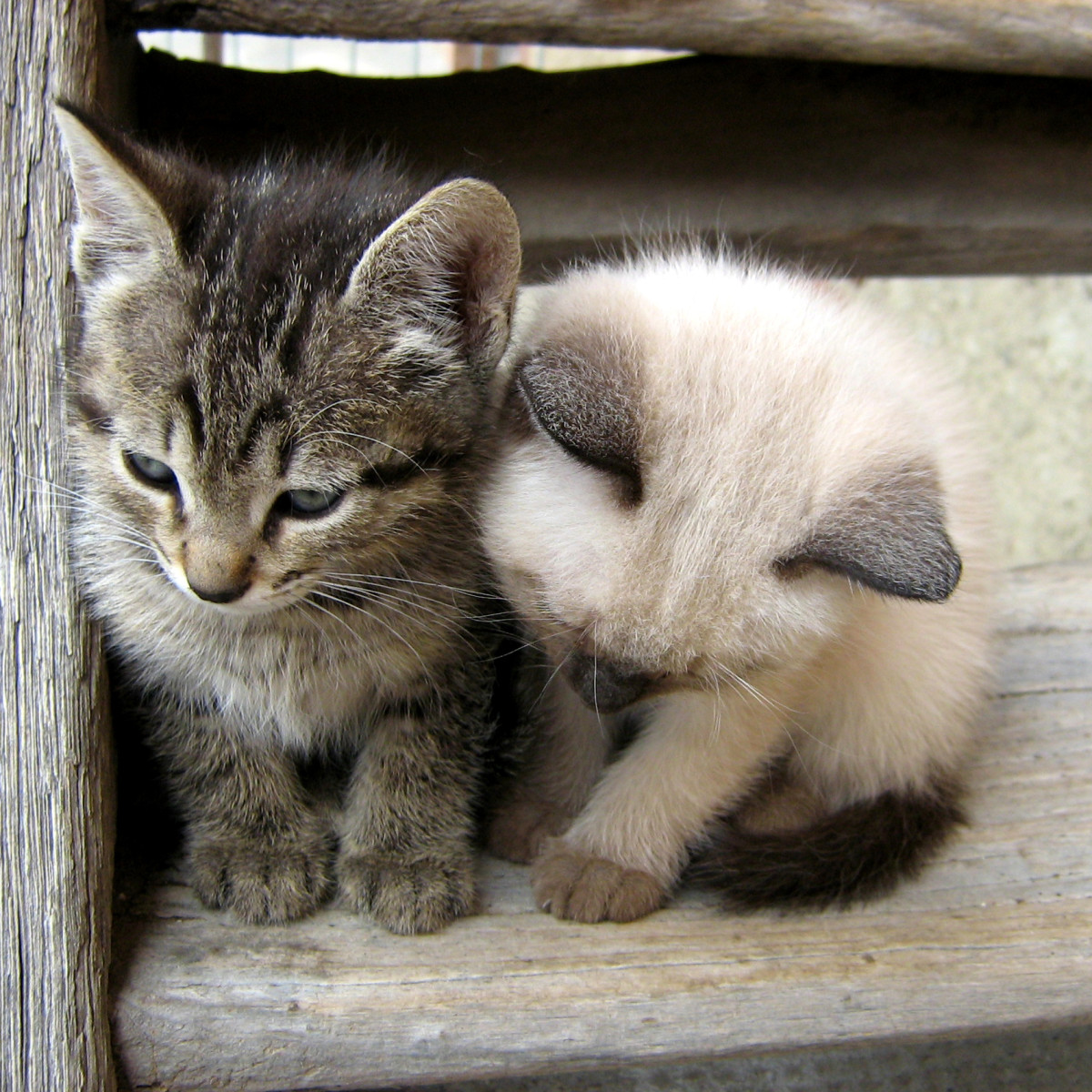Updated date:

# Cat Mathematics: Cat Stacking, Catenary Cables, & Cat Piles

Chris has a Master's degree in engineering and uses his knowledge to write about a variety of topics from an analytical perspective.Who knew that cats and math would go so well together? Many of you reading this article have already read my first one about this subject (if not, I've provided a link at the bottom of the article). Previously, I wrote about how many cats would inside or on top of a variety of things. I also had some fun discovering what a cat's terminal velocity was. Well that article was so well received (and I had so much fun writing it) that I'm back for a second installment. This time around the problems will be a bit more complicated. A few will even involve some fundamental equations of structural and geotechical engineering. I will explore a cat stacking problem, the catenary cable, and some properties of cat piles.

## Cat Stacking

In this first series of problems we answer some burning questions about cat stacking. More specifically I am interested in how many cats tall various things are. Additionally, I also wanted to answer this question while considering the effects compression on the cats due to the weight of the animals above them. To account for compression of the cat we must first develop a relationship between an applied force (stress) and the amount of compression (strain). From a fundamental structural engineering concept, this relationship is:Where Δh is the overall change in height of the stack of cats, n is the number of cats in the stack, m is the mass of a cat, h1 is the initial height of a single cat, E is the modulus of elasticity of a cat (Young's Modulus) , and A is the contact area (four paws) between a cat and the one above it.

For this problem we will need some engineering parameters of an average domestic cat.

m = 2.5 kg (5.5 lbs) - [Mass of one cat]

g = 9.81 m/s2 - [Acceleration due to Gravity]

h1 = 0.254m (10 inches) - [Height of one cat]

A = 0.00226m2 (3.5in2) - [Contact area for four paws]

E = 10,000,000 Pa (1,450 lbs/in2) - [Based on research of Young's Modulus for a variety of biological materials]

Calculating the number of cats required to reach a certain height while in a state of compression will require an iterative process. First, we need to estimate the number of cats in an uncompressed condition that are required to reach the height that we are looking for. Next, we will solve the equation to calculate the change in height of the stack of cats. This will enable us to calculate how many more cats we need to fill reach the required height. Next we can add those additional cats to the stack and repeat the calculation until the total height of the cat stack is equal to the height of the object you are looking for.

Fortunately, I have done all of the legwork for in a spreadsheet. The table below shows the number of cats required to reached a variety of heights for both compressed and non-compressed cats.

ObjectHeightNumber of CatsNumber of Cats (Compressed)Total Compression (m)Final Height (m)

Giraffe

18ft (5.5m)

22

23

0.01

5.84

Typical 2-Story House

26ft (8m)

31

32

0.01

8.12

Jeddah Light House

436ft (133m)

524

525

0.14

133.21

Great Pyramid

481ft (146m)

578

579

0.16

146.91

Gateway Arch

630ft (192m)

756

757

0.21

192.07

Eiffel Tower

986ft (301m)

1184

1186

0.33

300.92

Empire State Building

1,250ft (381m)

1501

1503

0.41

381.35

Petronas Towers

1,482ft (452m)

1779

1781

0.49

451.88

## "Cat"enary Cable (Chain of Cats)

In physics and geometry, a catenary is the curve that a hanging cable or chain makes under its own weight when supported only at its ends. Catenaries are seen almost everywhere in the urban environment. A prime example of a catenary shape are the cables hanging between telephone or power poles. The mathematics of the catenary shape has always intrigued me. Now that we are on the subject of cats, I wondered about properties of a hanging chain of these cute creatures. There are many questions that I could ask, but for now I am only interested in one: How far a chain of cats of a given length would sag if stretched between two standard 69kV power poles.CWanamaker

Before we solve this "cat"enary quandary we must define some parameters and assumptions. First, lets discuss the situation with the power poles. Typically, 69kV power lines are built on a mix of steel and wood poles measuring roughly 65ft (19.8m) tall. The distance between poles is usually between 220 feet to 400 feet. For this analysis we will assume that the distance is 300ft (91.5m). As far as cats are concerned we need to know their length and the distance of their overbite (because the cats' jaws will be clamped onto an adjacent cat's tail). From a previous article I wrote about the relative speed of animals, we know that an average cat's length from nose to tail is 2.5ft (0.762m). For this article we will assume that the overbite distance is 2.0inches (0.0508m). Therefore:

H1 = H2 = 19.8m - [Height of 69kv Poles]

D = 91.5m - [Distance between 69kv Poles]

L = n(l-B) - [Number of cats times the length of each cat minus it's overbite]

Now that we've defined some parameters we can finally set up the equations. There are four equations that are needed to help us solve this problems.The parameters a, b, & c in the above equations are parameters that define the catenary curve. You can find these by solving the last three equations as a system. Once you find these parameters you can use them in the first equation. Since the poles are of equal height, we know that the lowest part of the cable will be halfway between the two poles (D/2). Inserting this value for x in the first equation will give you the height of the cable above the ground. Subtracting the result from the height of the power pole will yield the distance that the cat chain is sagging.

I've spared you the legwork for this problem as well. The table below shows the number of cats and sag distances resulting from a chain of cats of varied lengths stretched between two standard 69kV power poles.

Number of CatsChain Length (m)Sag Distance (m)Height Above Ground (m)

129

91.74

48.10

16.90

131

93.17

52.81

12.19

133

94.59

55.62

9.38

135

96.01

57.86

7.14

137

97.43

59.79

5.21

139

98.86

61.53

3.47

141

100.28

63.13

1.87

143

101.70

64.62

0.38

144

102.41

65.34

-0.34

So from this analysis we can say that it would take between 143-144 cats linked together to form a chain long enough to stretch between two 65ft high power poles spaced 300ft apart and just touch the ground! What's more is that this chain of cats would weigh over 786.5 pounds!Captain Sprite, CC-BY-SA-2.5, via Wikimedia Commons

## The Cat Pile

For my final mathematics problem in this article I'd like to talk about cat piles. A cat pile is very much like a traditional dog pile except that it involves our favorite feline friends instead. To form a cat pile you only need at least three cats. Any less than that and you would only have a cat stack instead.

I could write an entire article on the subject of cat piles (and someday I might), but for now I want to focus on one question. What angle forms between the horizontal plane and the slope of a cat pile when the cats are simply poured from a container? In other words, what is the angle of repose for a cat pile?

In real life the angle of repose for most materials is actually measured in a lab directly rather than calculated based on the substance's physical properties. Obviously when dealing with cats we can't do that (they're much too fast anyway). With that said, we can approximate the angle of repose by assuming that for a pile of cats it is the same as the friction angle. We can simulate this using the following relationship.where, φ is the internal friction angle, σs is shear stress and σn is normal stress.

These two stresses are related to one another through what's called the coefficient of static friction (for non-moving objects). The relationship between normal and shear stress is given below:Making a substitution you get the final equation:Thus, the friction angle (and thus the angle of repose) is exclusively dependent upon the coefficient of static friction between two cats. Unfortunately the Internet does not appear to contain this value (get on it fellow nerds!) so we will have to approximate it using similar materials. Based on my research, a variety of fabrics seem to have a static coefficient of friction of approximately 0.4. Therefore, that's what we will use for this analysis.

Now solving the equation using a static coefficient of friction of 0.4, the friction angle (and thus the angle of repose) for a cat pile is computed to be: 21.8 degrees.

## Note:

No cats were harmed in the making of this article. The scenarios presented are not meant to resemble real life events and any similarities to such are purely coincidental.

© 2014 Christopher Wanamaker

## Comments

Christopher Wanamaker (author) from Arizona on March 24, 2014:

Shinkicker - Thanks for stopping by and leaving such a nice comment. I appreciate it. As far as cats turning on a light bulb, well, if you have Handycat by your side then you will only need one! Here is a picture of Handycat: http://imgur.com/hUwJe

Shinkicker from Scotland on March 24, 2014:

A true work of genius, you are a pioneer sir. I don't understand all the number crunching, or any of it probably, but I'm sure it makes sense.

But! How many cats does it take to change a lightbulb I wonder?

Voted up and awesome